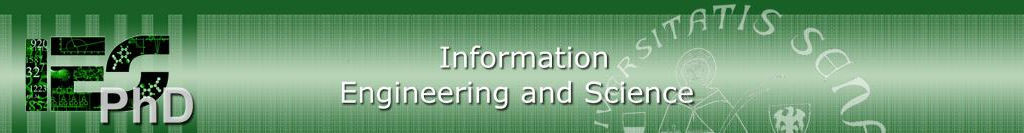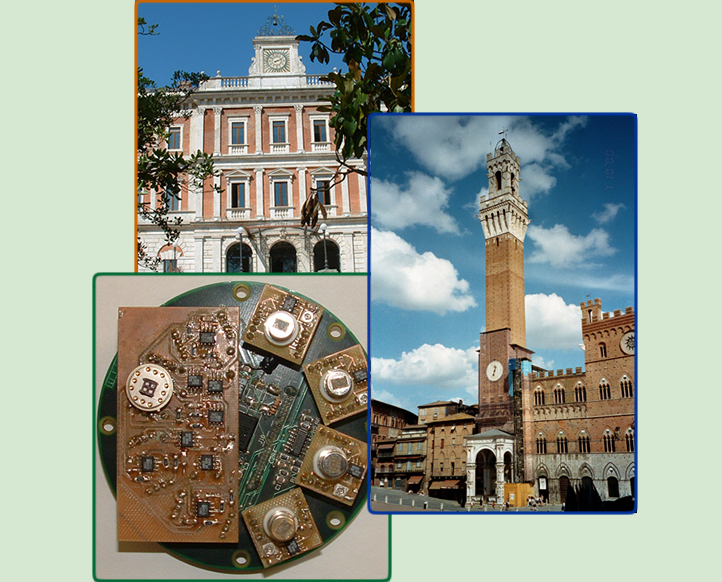Coordinator: Prof. Antonio Vicino University of SienaHome |  DIISM |   | Login Privacy e Cookie policy

InfoHow to applyInformation for PhD   studentsInternal RulesStructurePhD BoardResearch linesMathematical Logics

 Prof. Franco Montagna Università degli Studi di SienaDipartimento di Scienze Matematiche ed Informatiche Course Type Type A Calendar April 10: from 10 to 13 and from 14 to 15,30 (F. Montagna); (aim of the course; classical propositional logic) April 11: from 10 to 13 and from 14 to 15,30 (F. Montagna); (classical predicate logic) April 16: from 11 to 13 and from 14 to 16 (A. Sorbi); (the notion of computable function) April 17: from 11,30 to 13 and from 14 to 16,30 (A. Sorbi); (undecidable problems-incompleteness theorems) April 18: from 11,30 to 13 and from 14 to 16,30 (A. Sorbi); (some basic notions of computational complexity) April 19: from 10 to 13 and from 14 to 15,30 (F.Montagna); (automated deduction; some basic notions about logic programming) April 20: from 10 to 13 and from 14 to 15,30 (F. Montagna) (some non-classical logics) Room Program Part I. Propositional Calculus. Semantics and logical calculi. Sequent Calculus. Propositional resolution. Completeness. Resolution for Horn clauses and Krom clauses. First Order Calculus. Sequent Calculus. An outline of the Completeness Theorem for the first order. First order Resolution. Unification. Lifting Lemma. Completeness of Resolution. (10 hours, Franco Montagna.) Part II. The notion of a computable function. Turing machines and partial recursive functions. Decidable sets and undecidable sets. Undecidability in logic: The incompleteness theorems of Gödel and Church. Efficient computations. The classes P, NP, and co-NP. NP-completeness. Examples of NP-complete problems. Languages and their classification. The Chomsky Hierarchy. (15 hours, Andrea Sorbi.) Part III. A short excursus in some nonclassical logics with applications to computer science. Intuitionistic logic, and constructive mathematics. Kripke semantics. Semantics of proofs and types (outlines). Fuzzy logics. Semantics of t-norms. Applications: probability, Ulam games with lies, and fuzzy control (outlines). (5 hours, Franco Montagna.)

Courses2020-20212019-20202018-20192017-2018Previous yearsTeachersPoster DayEuropean School of   AntennasPhD Courses UnipiPhD Courses UnipgPhD Students/AlumniPhD studentsAlumniOther PhD programsDip. Ingegneria dell'Informazione e Scienze Matematiche - Via Roma, 56 53100 SIENA - Italy